# A quick tour of qcc

## Introduction

`qcc` is a contributed R package for statistical quality control charts which provides:

• Shewhart quality control charts for continuous, attribute and count data
• Cusum and EWMA charts
• Operating characteristic curves
• Process capability analysis
• Pareto chart and cause-and-effect chart
• Multivariate control charts.

This document gives a quick tour of `qcc` (version 2.7) functionalities. It was written in R Markdown, using the knitr package for production.

Further details are provided in the following paper:

Scrucca, L. (2004) qcc: an R package for quality control charting and statistical process control. R News 4/1, 11-17.

For a nice blog post discussing the `qcc` package, in particular how to implement the Western Eletric Rules (WER), see http://blog.yhathq.com/posts/quality-control-in-r.html.

``library(qcc)``

## Shewhart charts

### x-bar chart

``````data(pistonrings)
diameter = with(pistonrings, qcc.groups(diameter, sample))
``````##     [,1]   [,2]   [,3]   [,4]   [,5]
## 1 74.030 74.002 74.019 73.992 74.008
## 2 73.995 73.992 74.001 74.011 74.004
## 3 73.988 74.024 74.021 74.005 74.002
## 4 74.002 73.996 73.993 74.015 74.009
## 5 73.992 74.007 74.015 73.989 74.014
## 6 74.009 73.994 73.997 73.985 73.993``````
``q1 = qcc(diameter[1:25,], type="xbar", newdata=diameter[26:40,])``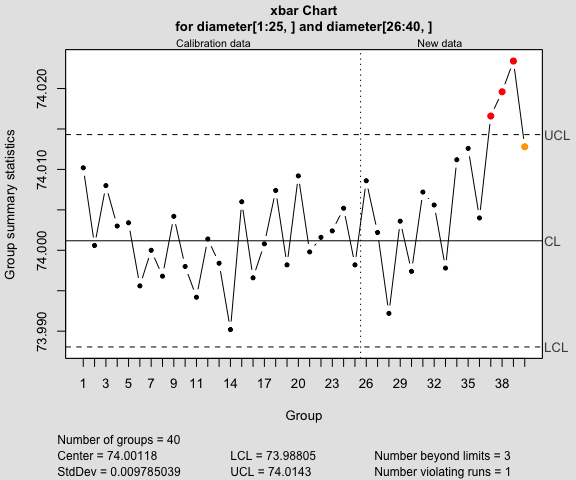``plot(q1, chart.all=FALSE)``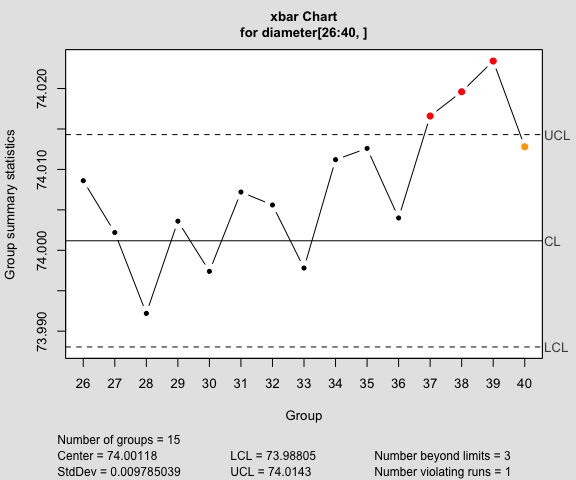``plot(q1, add.stats=FALSE)``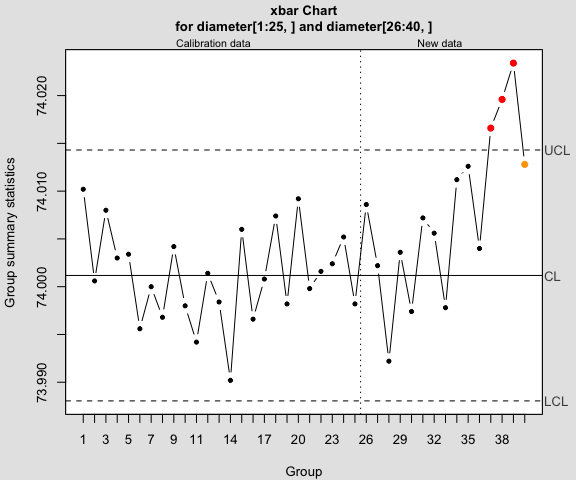``````q1 = qcc(diameter[1:25,], type="xbar", newdata=diameter[26:40,],
confidence.level=0.99)``````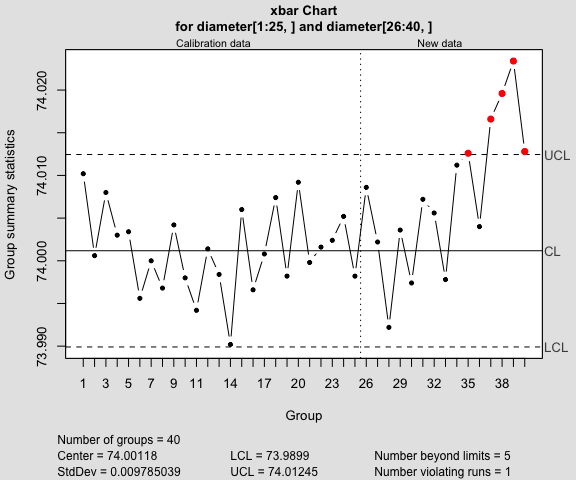Add warning limits at 2 std. deviations:

``````q1 = qcc(diameter[1:25,], type="xbar", newdata=diameter[26:40,], plot=FALSE)
(warn.limits = limits.xbar(q1\$center, q1\$std.dev, q1\$sizes, 2))``````
``````##       LCL      UCL
##  73.99242 74.00993``````
``````plot(q1, restore.par = FALSE)
abline(h = warn.limits, lty = 3, col = "chocolate")``````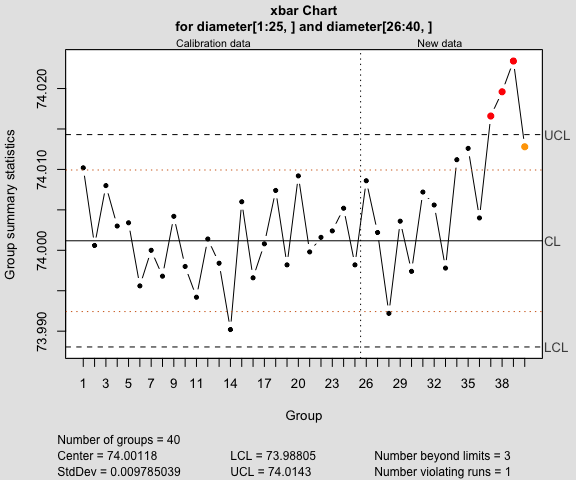### R chart

``q2 = qcc(diameter[1:25,], type="R")``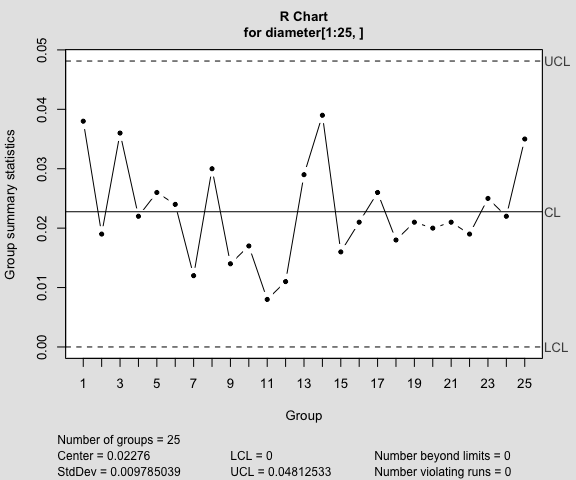``summary(q2)``
``````##
## Call:
## qcc(data = diameter[1:25, ], type = "R")
##
## R chart for diameter[1:25, ]
##
## Summary of group statistics:
##    Min. 1st Qu.  Median    Mean 3rd Qu.    Max.
## 0.00800 0.01800 0.02100 0.02276 0.02600 0.03900
##
## Group sample size:  5
## Number of groups:  25
## Center of group statistics:  0.02276
## Standard deviation:  0.009785039
##
## Control limits:
##  LCL        UCL
##    0 0.04812533``````
``q3 = qcc(diameter[1:25,], type="R", newdata=diameter[26:40,])``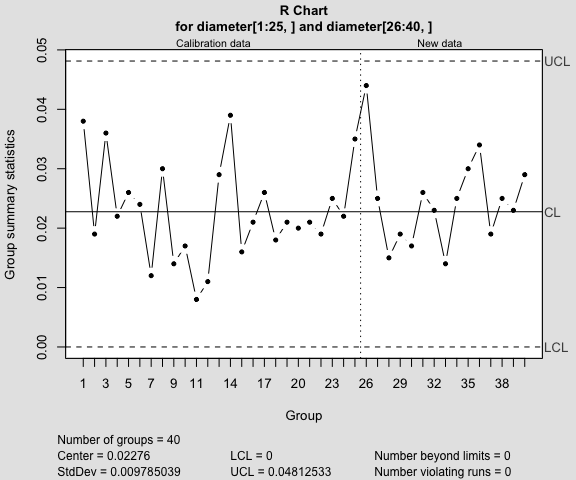``summary(q3)``
``````##
## Call:
## qcc(data = diameter[1:25, ], type = "R", newdata = diameter[26:40,     ])
##
## R chart for diameter[1:25, ]
##
## Summary of group statistics:
##    Min. 1st Qu.  Median    Mean 3rd Qu.    Max.
## 0.00800 0.01800 0.02100 0.02276 0.02600 0.03900
##
## Group sample size:  5
## Number of groups:  25
## Center of group statistics:  0.02276
## Standard deviation:  0.009785039
##
## Summary of group statistics in diameter[26:40, ]:
##       Min.    1st Qu.     Median       Mean    3rd Qu.       Max.
## 0.01400000 0.01900000 0.02500000 0.02453333 0.02750000 0.04400000
##
## Group sample size:  5
## Number of groups:  15
##
## Control limits:
##  LCL        UCL
##    0 0.04812533``````

### S chart

``q4 = qcc(diameter[1:25,], type="S")``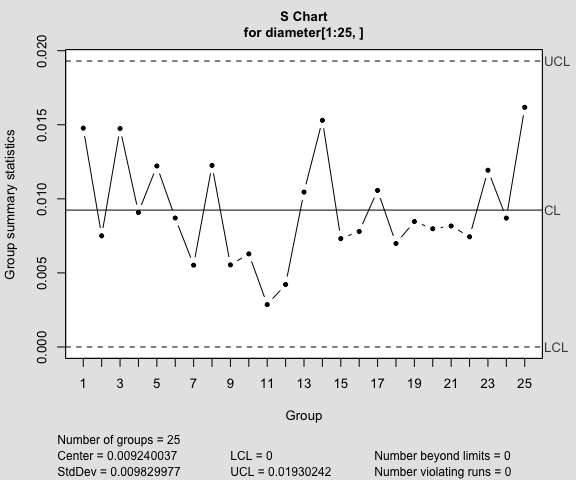``summary(q4)``
``````##
## Call:
## qcc(data = diameter[1:25, ], type = "S")
##
## S chart for diameter[1:25, ]
##
## Summary of group statistics:
##        Min.     1st Qu.      Median        Mean     3rd Qu.        Max.
## 0.002863564 0.007314369 0.008467585 0.009240037 0.011928956 0.016177144
##
## Group sample size:  5
## Number of groups:  25
## Center of group statistics:  0.009240037
## Standard deviation:  0.009829977
##
## Control limits:
##  LCL        UCL
##    0 0.01930242``````
``q5 = qcc(diameter[1:25,], type="S", newdata=diameter[26:40,])``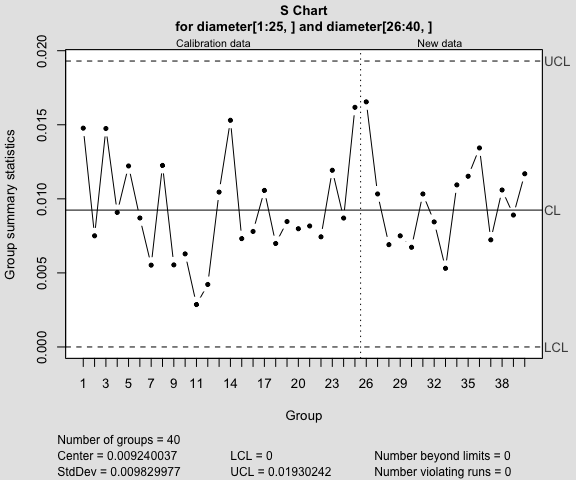``summary(q5)``
``````##
## Call:
## qcc(data = diameter[1:25, ], type = "S", newdata = diameter[26:40,     ])
##
## S chart for diameter[1:25, ]
##
## Summary of group statistics:
##        Min.     1st Qu.      Median        Mean     3rd Qu.        Max.
## 0.002863564 0.007314369 0.008467585 0.009240037 0.011928956 0.016177144
##
## Group sample size:  5
## Number of groups:  25
## Center of group statistics:  0.009240037
## Standard deviation:  0.009829977
##
## Summary of group statistics in diameter[26:40, ]:
##        Min.     1st Qu.      Median        Mean     3rd Qu.        Max.
## 0.005310367 0.007367603 0.010329569 0.009761757 0.011232319 0.016546903
##
## Group sample size:  5
## Number of groups:  15
##
## Control limits:
##  LCL        UCL
##    0 0.01930242``````

### Variable control limits

``````out = c(9, 10, 30, 35, 45, 64, 65, 74, 75, 85, 99, 100)
diameter2 = with(pistonrings, qcc.groups(diameter[-out], sample[-out]))
summary(qcc(diameter2[1:25,], type="xbar"))``````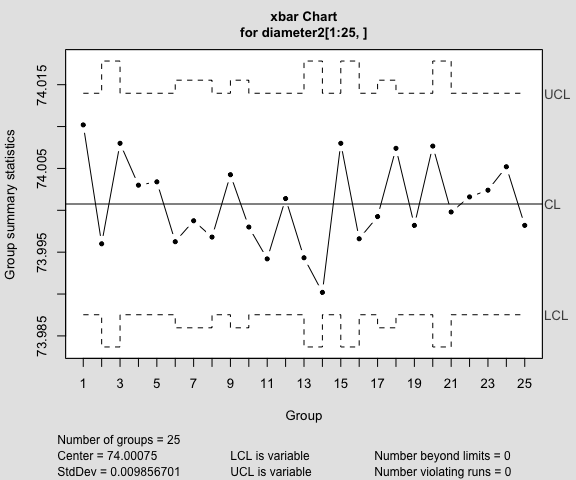``````##
## Call:
## qcc(data = diameter2[1:25, ], type = "xbar")
##
## xbar chart for diameter2[1:25, ]
##
## Summary of group statistics:
##     Min.  1st Qu.   Median     Mean  3rd Qu.     Max.
## 73.99020 73.99680 73.99980 74.00076 74.00425 74.01020
##
## Summary of group sample sizes:
##   sizes  3 4  5
##   counts 4 4 17
##
## Number of groups:  25
## Center of group statistics:  74.00075
## Standard deviation:  0.009856701
##
## Control limits:
##          LCL      UCL
##     73.98753 74.01398
##     73.98368 74.01782
## ...
##     73.98753 74.01398``````
``summary(qcc(diameter2[1:25,], type="R"))``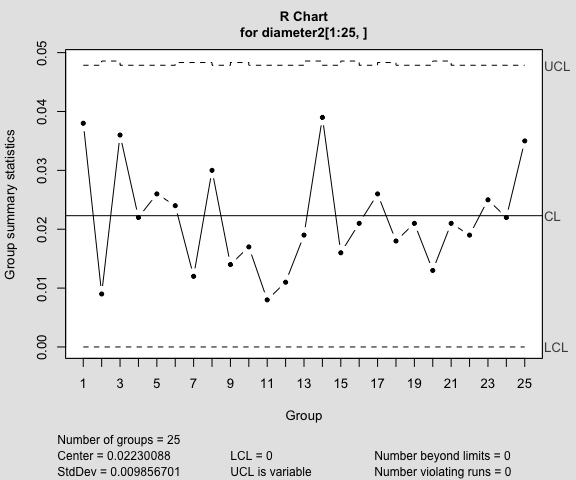``````##
## Call:
## qcc(data = diameter2[1:25, ], type = "R")
##
## R chart for diameter2[1:25, ]
##
## Summary of group statistics:
##    Min. 1st Qu.  Median    Mean 3rd Qu.    Max.
## 0.00800 0.01600 0.02100 0.02168 0.02600 0.03900
##
## Summary of group sample sizes:
##   sizes  3 4  5
##   counts 4 4 17
##
## Number of groups:  25
## Center of group statistics:  0.02230088
## Standard deviation:  0.009856701
##
## Control limits:
##     LCL        UCL
##       0 0.04785198
##       0 0.04857007
## ...
##       0 0.04785198``````

### p and np charts

``````data(orangejuice)
q1 = with(orangejuice,
qcc(D[trial], sizes=size[trial], type="p"))``````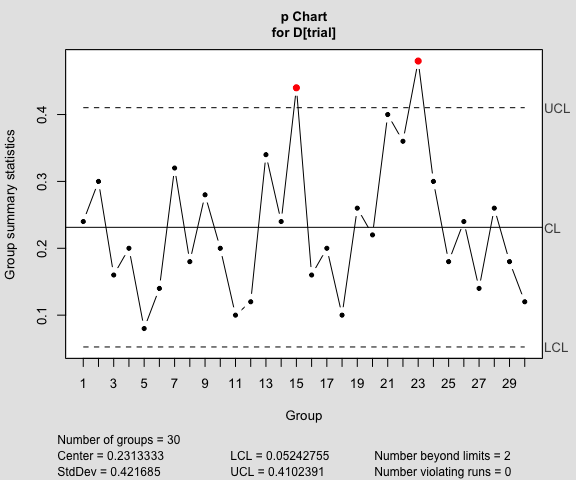``summary(q1)``
``````##
## Call:
## qcc(data = D[trial], type = "p", sizes = size[trial])
##
## p chart for D[trial]
##
## Summary of group statistics:
##      Min.   1st Qu.    Median      Mean   3rd Qu.      Max.
## 0.0800000 0.1600000 0.2100000 0.2313333 0.2950000 0.4800000
##
## Group sample size:  50
## Number of groups:  30
## Center of group statistics:  0.2313333
## Standard deviation:  0.421685
##
## Control limits:
##            LCL       UCL
##     0.05242755 0.4102391
##     0.05242755 0.4102391
## ...
##     0.05242755 0.4102391``````
``````q2 = with(orangejuice,
qcc(D[trial], sizes=size[trial], type="np"))``````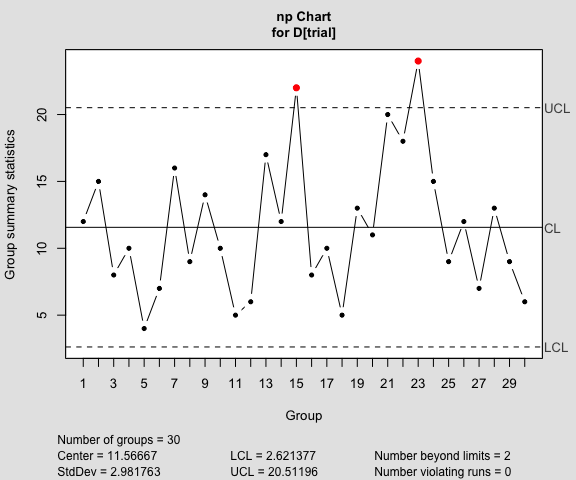``summary(q2)``
``````##
## Call:
## qcc(data = D[trial], type = "np", sizes = size[trial])
##
## np chart for D[trial]
##
## Summary of group statistics:
##     Min.  1st Qu.   Median     Mean  3rd Qu.     Max.
##  4.00000  8.00000 10.50000 11.56667 14.75000 24.00000
##
## Group sample size:  50
## Number of groups:  30
## Center of group statistics:  11.56667
## Standard deviation:  2.981763
##
## Control limits:
##       LCL      UCL
##  2.621377 20.51196``````

Remove out-of-control points (see `help(orangejuice)` for the reasons):

``````inc = setdiff(which(orangejuice\$trial), c(15,23))
q2 = with(orangejuice,
qcc(D[inc], sizes=size[inc], type="p",
newdata=D[!trial], newsizes=size[!trial]))``````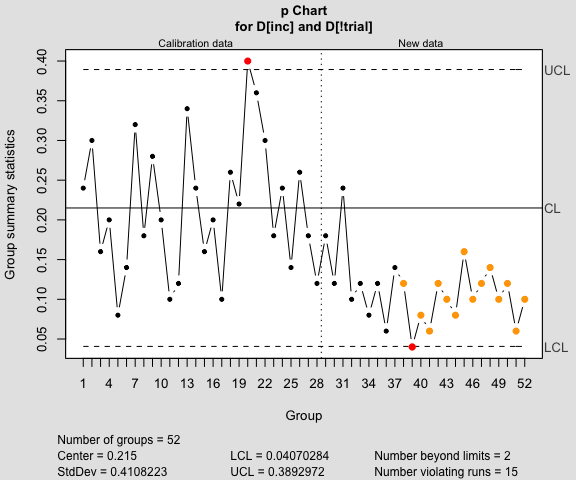``````data(orangejuice2)
q1 = with(orangejuice2,
qcc(D[trial], sizes=size[trial], type="p",
newdata=D[!trial], newsizes=size[!trial]))``````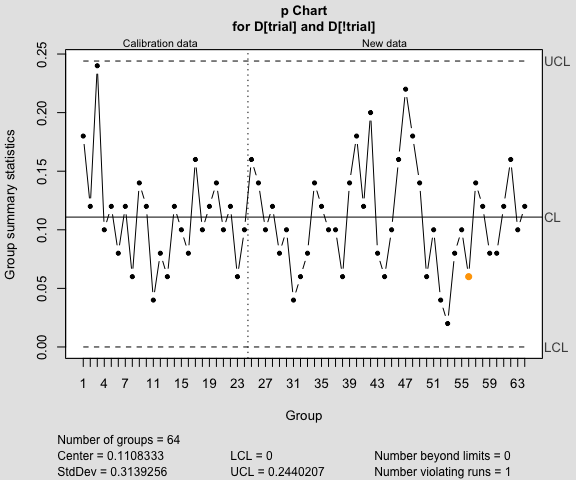``summary(q1)``
``````##
## Call:
## qcc(data = D[trial], type = "p", sizes = size[trial], newdata = D[!trial],     newsizes = size[!trial])
##
## p chart for D[trial]
##
## Summary of group statistics:
##      Min.   1st Qu.    Median      Mean   3rd Qu.      Max.
## 0.0400000 0.0800000 0.1100000 0.1108333 0.1200000 0.2400000
##
## Group sample size:  50
## Number of groups:  24
## Center of group statistics:  0.1108333
## Standard deviation:  0.3139256
##
## Summary of group statistics in D[!trial]:
##    Min. 1st Qu.  Median    Mean 3rd Qu.    Max.
##   0.020   0.080   0.100   0.109   0.140   0.220
##
## Group sample size:  50
## Number of groups:  40
##
## Control limits:
##     LCL       UCL
##       0 0.2440207
##       0 0.2440207
## ...
##       0 0.2440207``````

### c and u charts

``````data(circuit)
q1 = with(circuit,
qcc(x[trial], sizes=size[trial], type="c"))``````